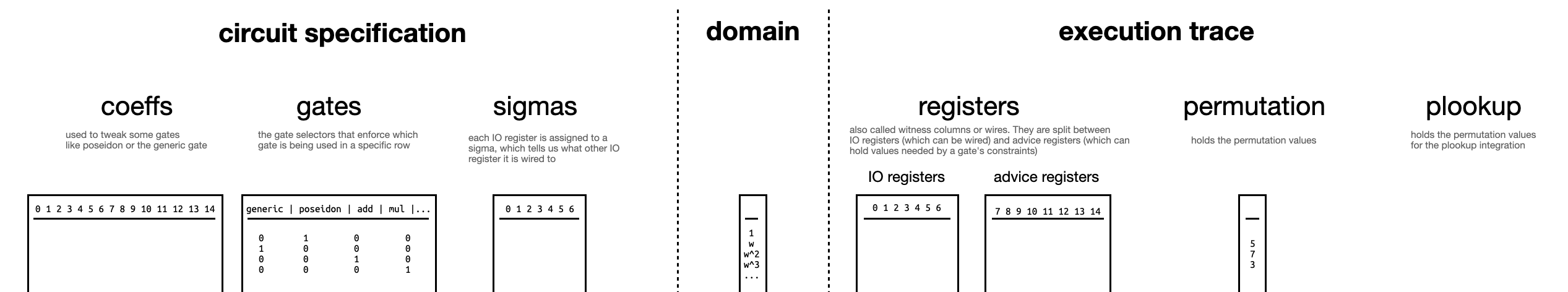# Overview

The proof system in Mina is a variant of . To understand , you can refer to our series of videos on the scheme. In this section we explain our variant, called kimchi.

kimchi is not formally a zk-SNARK, as it is not succinct in the proof size. zk-SNARKs must have a proof size where n is the number of gates in the circuit. In kimchi, due to the Bootleproof polynomial commitment approach, our proofs are where is the maximum degree of the committed polynomials (in the order of the number of rows). In practice, our proofs are in the order of dozens of kilobytes. Note that kimchi is a zk-SNARK in terms of verification complexity ()) and the proving complexity is quasilinear () – recall that the prover cannot be faster than linear.

Essentially what allows you to do is to, given a program with inputs and outputs, take a snapshot of its execution. Then, you can remove parts of the inputs, or outputs, or execution trace, and still prove to someone that the execution was performed correctly for the remaining inputs and outputs of the snapshot. There are a lot of steps involved in the transformation of “I know an input to this program such that when executed with these other inputs it gives this output” to “here’s a sequence of operations you can execute to convince yourself of that”.

At the end of this chapter, you will understand that the protocol boils down to filling a number of tables (illustrated in the diagram below): tables that specify the circuit, and tables that describes an execution trace of the circuit (given some secret and public inputs).